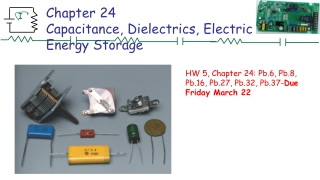Download PresentationChapter 24 Capacitance, Dielectrics, Electric Energy Storage# Chapter 24 Capacitance, Dielectrics, Electric Energy Storage - PowerPoint PPT Presentation

Download Presentation##### Chapter 24 Capacitance, Dielectrics, Electric Energy Storage

Download Policy: Content on the Website is provided to you AS IS for your information and personal use and may not be sold / licensed / shared on other websites without getting consent from its author. While downloading, if for some reason you are not able to download a presentation, the publisher may have deleted the file from their server.

- - - - - - - - - - - - - - - - - - - - - - - - - - - E N D - - - - - - - - - - - - - - - - - - - - - - - - - - -
##### Presentation Transcript

1. Chapter 24Capacitance, Dielectrics, Electric Energy Storage HW 5, Chapter 24: Pb.6, Pb.8, Pb.16, Pb.27, Pb.32, Pb.37-Due Friday March 22

2. 24-1 Capacitors A capacitor consists of two conductors that are close but not touching. A capacitor has the ability to store electric charge.

3. 24-1 Capacitors Parallel-plate capacitor connected to battery. (b) is a circuit diagram.

4. 24-1 Capacitors When a capacitor is connected to a battery, the charge on its plates is proportional to the voltage: The quantity C is called the capacitance. Unit of capacitance: the farad (F): 1 F = 1 C/V.

5. Problem 5 5. (II) A 7.7µF capacitor is charged by a 125-V battery (Fig. 24–20a) and then is disconnected from the battery. When this capacitor (C1) is then connected (Fig. 24–20b) to a second (initially uncharged) capacitor, the final voltage on each capacitor is 15V. What is the value of C2 [Hint: Charge is conserved.]

6. Problem 7 7.(II) It takes 15 J of energy to move a 0.20-mC charge from one plate of a 15µF capacitor to the other. How much charge is on each plate?

7. Problem 17 17. (II) How strong is the electric field between the plates of a 0.80µF air-gap capacitor if they are 2.0 mm apart and each has a charge of 92µC?

8. 24-2 Determination of Capacitance Capacitors are now made with capacitances of 1 farad or more, but they are not parallel-plate capacitors. Instead, they are activated carbon, which acts as a capacitor on a very small scale. The capacitance of 0.1 g of activated carbon is about 1 farad. Some computer keyboards use capacitors; depressing the key changes the capacitance, which is detected in a circuit.

9. 24-2 Determination of Capacitance

10. Question While a parallel plate capacitor is attached to a battery, the plates are separated a little more so that +Q -Q a) E increases and Q decreases b) E remains constant and Q increases c) E remains constant and Q decreases d) E decreases and Q decreases E V

11. Question While a parallel plate capacitor is attached to a battery, the plates are separated a little more so that +Q -Q a) E increases and Q decreases b) E remains constant and Q increases c) E remains constant and Q decreases d) E decreases and Q decreases E • C and Q decrease V • Q and E decrease

12. 24-2 Determination of Capacitance Example 24-1: Capacitor calculations. (a) Calculate the capacitance of a parallel-plate capacitor whose plates are 20 cm × 3.0 cm and are separated by a 1.0-mm air gap. (b) What is the charge on each plate if a 12-V battery is connected across the two plates? (c) What is the electric field between the plates? (d) Estimate the area of the plates needed to achieve a capacitance of 1 F, given the same air gap d.

13. 24-2 Determination of Capacitance Example 24-2: Cylindrical capacitor. A cylindrical capacitor consists of a cylinder (or wire) of radius Rb surrounded by a coaxial cylindrical shell of inner radius Ra. Both cylinders have length which we assume is much greater than the separation of the cylinders, so we can neglect end effects. The capacitor is charged (by connecting it to a battery) so that one cylinder has a charge +Q (say, the inner one) and the other one a charge –Q. Determine a formula for the capacitance.